逆矩阵

逆矩阵是什么？8 × (1/8) = 1

A × A-1 = I

(1/8) × 8 = 1
A-1 × A = I

单位矩阵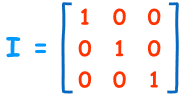3x3 单位矩阵

• 它是个"方形"矩阵（相同数目的行和列），
• 在对角线是 1，在其他位置是 0
• 符号是大写字母 I

定义

A的 逆（矩阵）是 A-1，仅当：

A × A-1 = A-1 × A = I

2x2 矩阵

2x2 矩阵的逆是：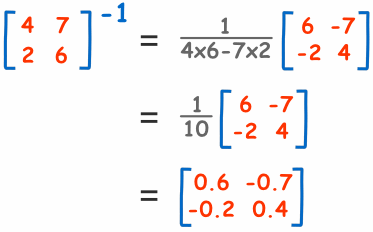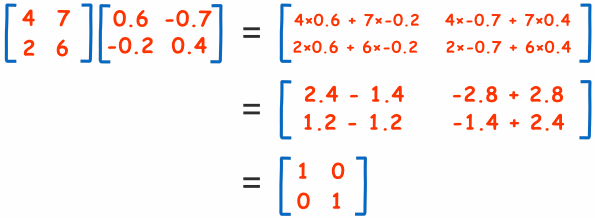A-1 × A = I 是对的。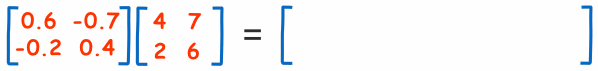我们为什么需要逆矩阵？

假设我们不能除以数字。。。。。。

。。。。。。那我我们怎样"把10个苹果分给2个人"呢？

10 × 0.5 = 5

XA = B

XAA-1 = BA-1

XI = BA-1

X = BA-1

实例：公交车与地铁XA = B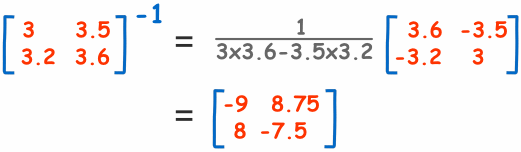X = BA-1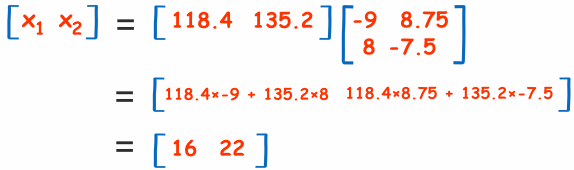次序是重要的

AX = B

A-1AX = A-1B

IX = A-1B

X = A-1B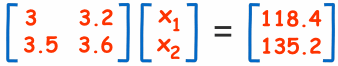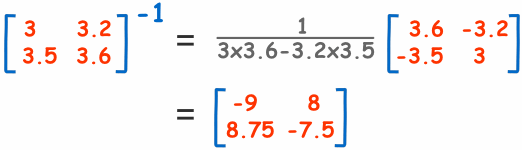X = A-1B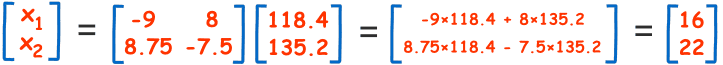可能没有逆矩阵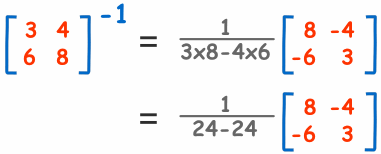24-24？ 等于 0， 1/0 是未定义的

（假想在公交车例子里，地铁的车费全是比公交车贵一半：我们便不能找出大人和小孩的分别。一定要有某些东西来使他们不同，我们才可以算出小孩和大人的数量。）

结论

• A 的逆矩阵是 A-1 仅当 A × A-1 = A-1 × A = I
• 求 2x2 矩阵的逆矩阵： 调换 a 和 d 的位置，把 负号 放在 b 和 c 前面，然后全部除以 矩阵的行列式 （ad-bc）。
• 有时候一个矩阵是没有逆矩阵的